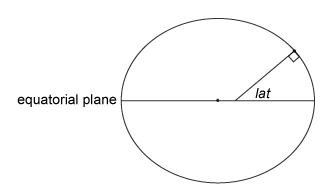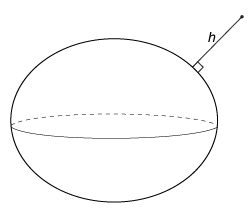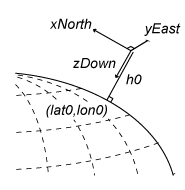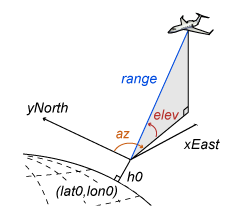## Comparison of 3-D Coordinate Systems

Coordinate systems represent position on the Earth using coordinates. Mapping Toolbox™ functions transform coordinates between Earth-centered Earth-fixed (ECEF), geodetic, east-north-up (ENU), north-east-down (NED), and azimuth-elevation-range (AER) systems.

Global systems such as ECEF and geodetic systems describe the position of an object using a triplet of coordinates. Local systems such as ENU, NED, and AER systems require two triplets of coordinates: one triplet describes the location of the origin, and the other triplet describes the location of the object with respect to the origin.

When you work with 3-D coordinate systems, you must specify an ellipsoid model that approximates the shape of the Earth. For more information about ellipsoid models, see Reference Spheroids. All of the sample coordinates on this page use the World Geodetic System of 1984 (WGS84).

### Earth-Centered Earth-Fixed Coordinates

An Earth-centered Earth-fixed (ECEF) system uses the Cartesian coordinates (X,Y,Z) to represent position relative to the center of the reference ellipsoid. The distance between the center of the ellipsoid and the center of the Earth depends on the reference ellipsoid.

• The positive X-axis intersects the surface of the ellipsoid at 0° latitude and 0° longitude, where the equator meets the prime meridian.

• The positive Y-axis intersects the surface of the ellipsoid at 0° latitude and 90° longitude.

• The positive Z-axis intersects the surface of the ellipsoid at 90° latitude and 0° longitude, the North Pole.For example, the ECEF coordinates of Parc des Buttes-Chaumont are (4198945 m, 174747 m, 4781887 m).

### Geodetic Coordinates

A geodetic system uses the coordinates (lat,lon,h) to represent position relative to a reference ellipsoid.

• lat, the latitude, originates at the equator. More specifically, the latitude of a point is the angle a normal to the ellipsoid at that point makes with the equatorial plane, which contains the center and equator of the ellipsoid. An angle of latitude is within the range [–90°, 90°]. Positive latitudes correspond to north and negative latitudes correspond to south.• lon, the longitude, originates at the prime meridian. More specifically, the longitude of a point is the angle that a plane containing the ellipsoid center and the meridian containing that point makes with the plane containing the ellipsoid center and prime meridian. Positive longitudes are measured in a counterclockwise direction from a vantage point above the North Pole. Typically, longitude is within the range [–180°, 180°] or [0°, 360°].• h, the ellipsoidal height, is measured along a normal of the reference spheroid. Coordinate transformation functions such as `geodetic2ecef` require you to specify h in the same units as the reference ellipsoid. You can change the units of the reference ellipsoid using the `LengthUnit` property. Terrain models typically supply data using orthometric height rather than ellipsoidal height. For information about calculating ellipsoidal height from orthometric height, see Find Ellipsoidal Height from Orthometric and Geoid Height.For example, the geodetic coordinates of Parc des Buttes-Chaumont are (48.8800°, 2.3831°, 124.5089 m).

### East-North-Up Coordinates

An east-north-up (ENU) system uses the Cartesian coordinates (xEast,yNorth,zUp) to represent position relative to a local origin. The local origin is described by the geodetic coordinates (lat0,lon0,h0). Note that the origin does not necessarily lie on the surface of the ellipsoid.

• The positive xEast-axis points east along the parallel of latitude containing lat0.

• The positive yNorth-axis points north along the meridian of longitude containing lon0.

• The positive zUp-axis points upward along the ellipsoid normal.For example, Montmartre has geodetic coordinates (48.8862°, 2.3343°, 174.5217 m). The ENU coordinates of Parc des Buttes-Chaumont with respect to Montmartre are (3579.4232 m, –688.3514 m, –51.0524 m).

### North-East-Down Coordinates

A north-east-down (NED) system uses the Cartesian coordinates (xNorth,yEast,zDown) to represent position relative to a local origin. The local origin is described by the geodetic coordinates (lat0,lon0,h0). Typically, the local origin of an NED system is above the surface of the Earth.

• The positive xNorth-axis points north along the meridian of longitude containing lon0.

• The positive yEast-axis points east along the parallel of latitude containing lat0.

• The positive zDown-axis points downward along the ellipsoid normal.An NED coordinate system is commonly used to specify location relative to a moving aircraft. In this application, the origin and axes of an NED system change continuously. Note that the coordinates are not fixed to the frame of the aircraft.

For example, an aircraft flying into Charles de Gaulle airport has geodetic coordinates (48.9978°, 2.7594°, 699.8683 m). The NED coordinates of the airport with respect to the plane are (1645.8313 m, –15677.1868 m, 555.8221 m).

### Azimuth-Elevation-Range Coordinates

An azimuth-elevation-range (AER) system uses the spherical coordinates (az,elev,range) to represent position relative to a local origin. The local origin is described by the geodetic coordinates (lat0,lon0,h0). Azimuth, elevation, and slant range are dependent on a local Cartesian system, for example, an ENU system.

• az, the azimuth, is the clockwise angle in the xEast-yNorth plane from the positive yNorth-axis to the projection of the object into the plane.

• elev, the elevation, is the angle from the xEast-yNorth plane to the object.

• range, the slant range, is the Euclidean distance between the object and the local origin.For example, a lidar sensor at the Charles de Gaulle airport has geodetic coordinates (48.0124°, 2.5451°, 163.4885 m). The AER coordinates of an airplane with respect to the sensor are (95.8314°, 1.8781°, 15773.1381 m).

### Tips

If you are transforming coordinates between ENU, NED, and AER systems with the same origin, then you do not need to specify a reference ellipsoid or the coordinates of the origin.